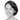# Basics of Pre- and Post-Money Option Pools

If you’ve never understood what’s a pre- and post-money option pool (aka the option pool shuffle), here’s your chance.

If you’ve never understood what’s a pre- and post-money option pool (aka. the option pool shuffle) here’s your chance. Get a piece of paper and a pencil, write down the formulas and don’t give up until the end of this post!

#### How it all started

Sheryl and Elon are two good friends who decided to co-found a company called SpaceBook (the social network for aspiring communities on Mars). Their initial cap table looks as below, with the total number of shares being 1 million:

Now, they want to raise a seed round. They manage to get a deal with an investor, Peter:

• Peter invests $500,000 • Everyone agrees on a pre-money valuation of$5 million

This means that Peter will receive 9.09% of the company. How do we know that?

• We know the share price:
$\text{PricePerShare} = \frac{\text{PreMoneyValuation}}{\text{PreMoneyShares}} = \frac{\text{\5 million}}{\text{1 million}} = \5$
• Peter will receive the following number of shares:
$\frac{\text{Peter’s investment}}{\text{PricePerShare}} = \text{100,000 shares}$
• The new total number of shares in the company:
$\text{PostMoneyShares} = \text{PreMoneyShares} + \text{100,000} = 1.1\text{ million}$
• Peter’s percentage:
$\frac{\text{PreMoneyShares}}{\text{PostMoneyShares}} = 9.09\%$

#### Option Pool

Now that Sheryl, Elon, and Peter have agreed on the basic deal, they start talking about reserving shares for employees, i.e. creating a stock option pool. There are basically two possibilities: a pre-money (aka investor friendly) or a post-money (aka founder friendly) option pool. The naming is a little confusing since different people use the naming pre or post for different purposes, so make sure you know if you are talking about an investor or founder friendly pool. I will explain in more details what each of them means below — for now, read on.

Let’s say we want to create an option pool of 10% (in the fully diluted cap table after the capital increase), i.e. the $\text{PoolShares}$ have to be equal to 10% of the total number of shares after the investment. In this case, the following equation is true:

$\text{(Sheryl + Elon+ Peter)’ shares} = \textbf{90\%} * (\text{(Sheryl + Elon+ Peter)’ shares} + \text{PoolShares})$

Simply reshuffling and isolating the terms, we have:

$\text{PoolShares} = \text{(Sheryl + Elon+ \textbf{Peter})’ shares} * \left( \frac{1}{90\%} - 1 \right)$

Think thoroughly about this, and once you understand it, just accept it and notice one important thing we’ll need later on: The $\text{PoolShares}$ depend on the number of Peter’s shares.

#### So, what is a post-money (founder friendly) option pool?

Very easy to calculate and understand. The round is done as explained above. Then an option pool of 10% is created, which dilutes everyone. In this case, Peter will end up with less than the planned 9.09%, after the pool is created.

#### What is a pre-money (investor friendly) option pool?

This is tricky to calculate because it involves equations that have to be solved numerically. And it’s founders-unfriendly because only the founders (Sheryl and Elon) get diluted. In which case would Sheryl and Elon go for a pre-money pool? In the event that Peter, for instance, says “A deal is a deal. With or without the option pool, I want to have my 9.09% for my $500,000 investment as agreed before this whole pool story!” This means, the shares created for the pool have to be created, so to say, before Peter invests in the company so that he does not get diluted. In other words, the price per share that Peter will pay will diminish: $\text{PricePerShare} = \frac{\text{PreMoneyValuation}}{\text{(Sheryl + Elon)’ shares}} \Longrightarrow \frac{\text{PreMoneyValuation}}{\text{(Sheryl + Elon)’ shares} + \textbf{PoolShares}}$ This again means, that if Peter gets a cheaper price per share, then he will get more shares for his$500,000 investment. An important detail to be noticed here: The number of Peter’s shares depends on the number of shares in the option pool!

By now, you should see that we have a “problem”: The number of shares in the option pool depends on the number of Peter’s shares because it has to represent exactly 10% of the total shares after the capital increase. On the other hand, the number of Peter’s shares also depends on the number of shares in the option pool because his stake also needs to be exactly 9.09% as agreed during the deal. This is why we need to solve this numerically which is what Ledgy’s round modeling does.

Additionally, if you want to compare scenarios and see what happens if you decide for post-money vs pre-money option pool for your startup, you can model it with the Ledgy app for free.

#### Still wondering if you should go for a pre- or post-money option pool?

As stated above, the post-money is friendlier for the founders. But if your investors insist on a pre-money pool, you can also, for example, negotiate the company’s valuation to be higher in order to reach a win-win situation.

Please reach out if anything’s unclear.

#### What's next?

Learn how to model a round by watching our webinar!

25 Feb 2019